# Fluid Flow and Heat Transfer in Porous Media and Post Heated Obstacle: Lattice Boltzmann Simulation

Fluid Flow and Heat Transfer in Porous Media and Post Heated Obstacle: Lattice Boltzmann Simulation

S. ChattiC. Ghabi A. Mhimid

Laboratory of Thermal and Energy Systems Studies, National Engineering School of Monastir, Monastir University, 5019 Monastir, Tunisia

Corresponding Author Email:
chatti_saida@yahoo.com
Page:
377-385
|
DOI:
https://doi.org/10.18280/ijht.340305
|
Accepted:
|
Published:
30 September 2016
| Citation

OPEN ACCESS

Abstract:

The purpose of this paper is to study heat transfer and fluid flow in porous media with and without obstacle. The double-population thermal lattice Boltzmann is applied. The validation of the accuracy of our numerical code is done. We resort to a comparison between the present and the numerical results reported in previous studies on benchmark problems (the generalized Poiseuille flow). Then, the problem of a fluid sheared in a porous media is studied using two approaches of LBM: generalized lattice Boltzmann equation (GLBE) and simplified lattice Boltzmann equation (SLBE). The comparison of these models is achieved at a specific node and cross-section. It includes many dynamic parameters and covers all over the medium. It is so important to conclude that the GLBE is more suitable to study heat transfer in porous media. In the second part, the heat transfer is calculated through a porous media with hot obstacle. The effect of the obstacle dimension and position is presented. The dependence of fluid flow and heat transfer to several parameters is studied. We conclude by showing that the model presented here is particularly well-suited to study heat and mass transfer in porous media with many applications.

Keywords:

Lattice Boltzmann equation (GLBE and SLBE), Porous media, Thermal incompressible flow, Convection, Hot obstacle.

1. Introduction

The heat transfer mechanism and the fluid flow in porous media are of crucial importance in many industrial and engineering fields such as chemical and petroleum engineering .

Understanding this phenomenon requires unrelentingly solving partial differential equations (PDE) (Navier-Stokes equations (NS)). The simulation is on three scales. For macroscopic one many methods of CFD are available such as finite difference (FDM). The microscopic scale is based on molecular dynamic. Recently lattice Boltzmann method (LBM) has appeared as an alternative computationally potent tool to simulate a Newtonian fluid flow especially in complex geometries [2, 3]. The basic idea of LBM is the kinetic theory of fluid motion; it's derived from the Boltzmann transport equation. In fact, the LBM simulation consists of registering the evolution of fluid particle in discretized space, speed and time. LBM received considerable attention thanks to many advantages: the convection operator of the kinetic equation is linear. It’s adapted to parallel processes computing and solving the Laplace equation is not necessary at each time step to satisfy the continuity equation. The Lattice Boltzmann method is used to model flow behavior in complex geometries thanks to its easy implementation under complex fluid–solid boundary conditions. In comparison with conventional CFD methods, the LBM has simple calculation procedure [4, 5]. The main advantage of this method is the simple implementation of boundary conditions. These benefits motive the simulation of non-compressible fluid flow in porous media. In fact, two models of LBM are proposed: Simplified lattice Boltzmann equation (SLBE) accounts of the viscous stress influence. It is based on Brinkman coefficient. This correction is valid for high porosity where the effect of Forchheimer term is undefined. Thus, studying non-compressible fluid flow according to the only Brinkman modification does not generate good agreement with the experimental results also ignoring the impact of Brinkman is not adequate for all conditions . It is necessary to combine the two coefficients to obtain equation that contains inertial and viscous effect. So the second model generalized lattice Boltzmann equation (GLBE) emerges. It is founded on the Brinkman-Forcheimer equation. This model considers the linear and nonlinear drag forces resulting of solid matrix in addition to viscous stress due to solid boundaries . Several researches were interested on the heat transfer in porous media and around obstacle. Indeed, the heat transfer and fluid flow in porous media bounded by two isothermal parallel plates was studied . The effect of parameters such as porosity, Reynolds and thermal diffusivity were investigated [9-13]. We are interested on the convection in a porous channel with fixed wall containing hot square due to its appearance in many scientific and industrial fields such as water filtration, catalytic converter and petroleum exploration and production.

In the present paper the lattice Boltzmann method is applied to study heat transfer in complex structure. Indeed, a new numerical code is developed to treat fluid flow and heat transfer in porous media. The paper contains three sections. Firstly, the computational method is defined. The theory aspect of the thermal D2Q9 model is described. The second part is devoted to the mathematical formalism modeling the fluid flow and the heat transfer in porous channel. The third part can be divided on subsection. The first one concerns the validation of the numerical code, which is done by the study of Poiseuille flow. The analysis of the root mean square error shows good agreement between the LBM results and the benchmark case. Then, we use the SLBE and GLBE to simulate the thermal injected fluid flow in porous channel. The third subsection is interested on the comparison of the LBM approaches. It is effectuated at different positions and for different dynamics parameters. For small Darcy and Reynolds number the two models coincide. We use the GLBE to study heat transfer and fluid behavior in porous plate. The streamline and the isotherms are shown at different thermodynamic parameters. Also, the temperature and the velocity profiles are treated.

Finally, we dealt the convection and the fluid behavior post obstacle placed inside porous media. The isotherms, the velocity field, the streamline and the pressure are studied for different parameters. The effect of the obstacle position and dimension is presented. The document describes the lattice Boltzmann approaches and shows its ability to treat easily complex problems. It offers a full study of convection and fluid flow in porous media which is important for several applications.

2. Lattice Boltzmann Method

The fundamental equation of the lattice Boltzmann method is clearly presented in many papers such as [5, 14] by:

$f\left(x+\delta t c_{i}, t+d t\right)_{i}-f(x, t)_{i}=\frac{f(x, t)_{i}-f(x, t)^{e q}}{\Gamma_{v}}+\delta t F_{i}$(1)

The relaxation time is $\Gamma_{v}$. It designs the time between two successive collisions. On the lattice space, it is defined as$\Gamma_{v}=\frac{3 u_{0} n_{y}}{\operatorname{Re}}$. $\mathbf{n}_{\mathbf{y}}$ is the lattice number on the characteristic length. For two dimensional, the nine-speed (D2Q9) LBE model is widely used. The discrete velocities and the weight are given by [14, 15]. The equilibrium distribution functions are given by the following equation :

$f_{i}^{e q}=\omega_{i} \rho\left[1+\frac{3 c_{i} u}{c^{2}}+\frac{9\left(c_{i} u\right)^{2}}{2 \varepsilon^{4}}-\frac{3 u^{2}}{2 \varepsilon c^{2}}\right]$(2)

The origin lattice is at rest and the others moving in different directions with different speed. The velocity vector indicates a lattice per unit step. Throughout the flow domain the particle mass is taken as unity uniformly. The macroscopic quantities are related to the distribution functions in many references such as . We assume that the viscous heat dissipation and the compression work generated by the pressure are negligible. The temperature is described by :

$g\left(x+c_{i} \delta t, t+\delta t\right)_{i}-g(x, t)_{i}=-\frac{g(x, t)_{i}-g(x, t)_{i}^{e q}}{\Gamma_{c}}$(3)

The thermal equilibrium distribution function is given by :

$\left\{\begin{array}{c}{g_{0}^{e q}=-\frac{2 \rho \Sigma u^{2}}{3 c^{2}}} \\ {g_{i=1,4}^{e q}=\frac{\rho \Sigma}{9}\left[\frac{3}{2}+\frac{3 c_{i} u}{2 c^{2}}+\frac{9\left(c_{i} u\right)^{2}}{2 c^{4}}-\frac{3 u^{2}}{2 c^{2}}\right]} \\ {g_{i=5,8}^{e q}=\frac{\rho \Sigma}{36}\left[3+\frac{6 c_{i} u}{c^{2}}+\frac{9\left(c_{i} u\right)^{2}}{2 c^{4}}-\frac{3 u^{2}}{2 c^{2}}\right]}\end{array}\right.$(4)

The temperature using the D2Q9 LBE model, is given by this equation

$T(x, t)=\frac{1}{\rho} \sum_{i=0}^{8} g(x, t)_{i}$(5)

3. Mathematical Equations and LBM Implementation

In porous media the equations are given in the representative elementary volume approach (REV). The Boussinesq approximation is applied. Indeed, the generalized model for non-compressible fluid flow and heat transfer is governed by the following equations [6, 17, 18]:

Continuity equation

$\nabla . u=0$(6)

Momentum equation

$\frac{\partial u}{\partial t}+(u . \nabla)\left(\frac{u}{\varepsilon}\right)=-\frac{1}{\rho} \nabla(\varepsilon p)+v_{e} \nabla^{2} u+F$(7)

Energy equation:

$\sigma \frac{\partial T}{\partial t}+\nabla \cdot(u T)=\alpha \nabla^{2} T$(8)

The relation between $\sigma$ and $\alpha$ is $\alpha=\sigma c_{S}^{2}\left(\Gamma_{c}-0.5\right)$. The bulk average pressure $P$ is expressed by:

$P=\frac{\rho c^{2}}{3 \varepsilon}$(9)

The effective viscosity $v_{e}$ is assumed to be equal to the viscosity $v,$ and $F$ represents the total external body force containing porous media resistance. The $F$ general expression is:

$F=-\frac{\varepsilon v}{K} u-\frac{\varepsilon F_{\varepsilon}}{\sqrt{K}}|u| u+\varepsilon G$(10)

The geometric function is $\mathrm{F}_{\varepsilon}=\frac{1.75}{\sqrt{150 \varepsilon^{3}}}$. The Brinkman term $\mathrm{U}_{\mathrm{e}} \nabla^{2} \mathrm{u}$ includes the viscous stress introduced by solid boundaries . This term is essential for flow with heat transfer and thin boundary layer. This restriction is called the Darcy-Brinkman extension. It defines the simplified lattice Boltzmann equation (SLBE) . The term $\frac{\varepsilon \mathrm{F}_{\varepsilon}}{\sqrt{\mathrm{K}}}|\mathrm{u}| \mathrm{u}$ refers to the nonlinear drag engendered by the solid matrix. It is the Forchheimer correction. This expression leads to generalized lattice Boltzmann equation. The forcing term is given by . To avoid the problem of the non-linearity we define a temporal velocity by the following relation [5, 6, 7, 16].

$\mathrm{u}=\frac{\mathrm{V}}{\mathrm{c}_{0}+\sqrt{\mathrm{c}_{0}^{2}+\mathrm{c}_{1}|\mathrm{v}|}}$(11)

4. Results and Discussion

4.1 Code validation: Poiseuille flow

The Poiseuille flow consists of a steady state flow in 2D channel of length $L$ and width $H$ filled with porous media characterized with porosity $\mathcal{E}$. The velocity $u$ verifies the following equation:

$\frac{v_{e}}{\varepsilon} \frac{\partial^{2} u}{\partial y^{2}}+G_{x}-\frac{v}{K} u-\frac{F_{\varepsilon}}{\sqrt{K}} u^{2}=0$(12)

The velocities on the walls ($\mathrm{u}(\mathrm{x}, 0)$ and $\mathrm{u}(\mathrm{x}, \mathrm{H})$) and the lateral velocity component is taken zero all over the domain. The bounce back and the periodic conditions are applied. They are respectively expressed as follow:

$f_{i}=f_{-i}, f\left(x_{1}, t\right)_{i}=f\left(x_{n}, t\right)_{i}$(13)

The inlet and the outlet positions are respectively $\mathrm{x}_{1}$ and $\mathrm{x}_{\mathrm{n}}$ The Reynolds number of Poiseuille flow $\mathrm{Re}$ is given by $\operatorname{Re}=\frac{\mathrm{Hu}_{0}}{v}$ where $\mathrm{u}_{0}$ refers to the peak velocity of the flow along the centerline of the channel [5-7]. The Darcy number is defined by $=\frac{\mathrm{K}}{\mathrm{H}^{2}}$ . The porosity is fixed to 0.1 and the Rayleigh number is equal to 0.1. Initially the velocity field is zero at each node. The resolution of equation (12) is made by applying the second order finite difference scheme with a uniform mesh (FDM). The grid size dependence is studied at different Reynolds number. The maximum velocity and pressure are compared. The results are shown in the Table1. The maximum change of using finer mesh is about 1.2%. The results are grid independent.  In the Figures 1 and 2 the velocity profiles for different values of Reynolds and Darcy are compared with Seta numerical results. These results are also verified by the FDM solutions. The Figure shows an agreement between the present results and the Seta work .

For more accuracy the root mean square error (RMSE) is calculated by the following equation:

$\sqrt{\frac{\sum_{1}^{\mathrm{z}}\left(\mathrm{M}_{\mathrm{LBM}}-\mathrm{M}_{\theta}\right)^{2}}{\mathrm{Z}}}$(14)

Figure 1 and figure 2 present a good agreement with Seta results for different Reynolds number and Darcy number of the velocity for poiseuille flow.

## figure_1.pngFigure 1. Velocity profile of the Poiseuille flow for different Reynolds number

Table 1. Grid dependence at different Reynolds number

 Reynolds Number Grids Size Max Horizontal Velocity Max Vertical Velocity Max pressure 1 80 × 80 0.891 9.78E-06 0.1111 100× 100 0.893 3.24E-05 0.1111 120×120 0.895 3.21E-05 0.1111 10 80 × 80 0.966 4.33E-04 0.1123 100× 100 0.967 2.70E-04 0.1123 120×120 0.969 3.95E-04 0.1123 0.01 80×80 0.561 1.96E-07 0.11111 100×100 0.5686 1.43E-07 0.11111 120×120 0.5687 7.64E-08 0.11111

## figure_2.pngFigure 2. Velocity profile of the Poiseuille flow for different Darcy number

The Table 2 gives the RMSE between the LBM results and the Seta work at different Reynolds.

Table 2. The RMSE at different Reynolds number

 Reynolds Number Root Mean Square Error 0,01 0,0164 1 0,0138 10 0,0425

The Table 3 gives the RMSE between the LBM results and the Seta work at different Darcy number.

Table 3. The root mean square error at different Darcy number

 Darcy Number Root Mean Square Error 0,001 0,019238 0,0001 0,029742 0,00001 0,029593

4.2 Thermal injected flows

The second case is a hot fluid sheared in a porous channel. It was injected normal to the shearing direction. The upper and lower plates are fixed and kept at a constant temperature respectively $\mathrm{T}_{\mathrm{h}}$ and $\mathrm{T}_{\mathrm{c}}$. On the inlet and the upper side the Zou and He work are applied to calculate the dynamic boundary. In the inlet, the unknown functions and the density are:

$\rho_{i n}=\frac{1}{1-u_{i n}}\left[f_{0}+f_{2}+f_{4}+2\left(f_{3}+f_{6}+f_{7}\right)\right]$(15)

$\left\{\begin{array}{c}{f_{1}=f_{3}+\frac{2}{3} \rho_{i n} u_{i n}} \\ {f_{7}=f_{5}-\frac{1}{2}\left(f_{2}-f_{4}\right)+\frac{1}{6} \rho_{i n} u_{i n}+\frac{1}{2} \rho_{i n} v_{i n}} \\ {f_{8}=f_{6}+\frac{1}{2}\left(f_{2}-f_{4}\right)+\frac{1}{6} \rho_{i n} u_{i n}+\frac{1}{2} \rho_{i n} v_{i n}}\end{array}\right.$(16)

On the bottom we use the bounce back conditions. For The thermal boundary conditions we resort to the Direchlet condition given by the following equation:

$g_{i}=T_{k}\left(\omega_{i}+\omega_{i+2}\right)-g_{i+2}$(17)

If the Forchheimer term is neglected the flow is governed at steady state by:

$\frac{v_{e}}{\varepsilon} \frac{\partial^{2} u}{\partial y^{2}}+G_{x}-\frac{v}{K} u=0$(18)

Where $G_{x}=-g_{0} \beta\left(T-T_{m}\right)+a_{y}$

The term $a_{y}$ is:

$a_{y}=\frac{v}{K} v_{0}-g_{0} \beta \Delta T\left[\frac{\exp \left(\frac{y v_{0}}{\alpha}\right)-1}{\exp \left(\frac{H v_{0}}{\alpha}\right)-1}\right]$(19)

The temperature satisfies the energy equation (equation (8)). At steady state the analytics solutions for velocity and temperature are expressed as follows:

$u=u_{0} \exp \left[r\left(\frac{y}{H}-1\right)\right] \frac{s h\left(\frac{C y}{H}\right)}{s h(c)}$(20)

$T=T_{h}+\Delta T \frac{\exp \left(\frac{P r * R e * y}{H}\right)-1}{\exp (\operatorname{Pr} * \operatorname{Re})-1}$(21)

$r, C$ and $\Delta T$ are respectively given by $r=\frac{R e}{2 \varepsilon} C=\frac{1}{2 \varepsilon} \sqrt{R e^{2}+\frac{4 \varepsilon}{D a}}$ and $\Delta T=T_{h}-T_{c}$.

The Rayleigh and Prandlt numbers are respectively 100 and 1. The porosity is equal to 0.7. The Darcy number is chosen to be 0.1 and the Reynolds number changes from 1 to 50. The grid independence of the results is established for different Reynolds numbers. The magnitude of the maximum $x$ and $y$-velocity along the horizontal centerline and the maximum of pressure with changing grid size are shown in the Table 4.

Table 4. Grid dependence at different Reynolds number

 Reynolds number Grid size Velocity (Y=0.98) Temperature (Y=0.98) 10 330×92 0.96 0.95 330×100 0.959 0.947 20 330×92 0.897 0.88 330×100 0.899 0.87

## figure_3a.png(a) Velocity profile

## figure_3b.png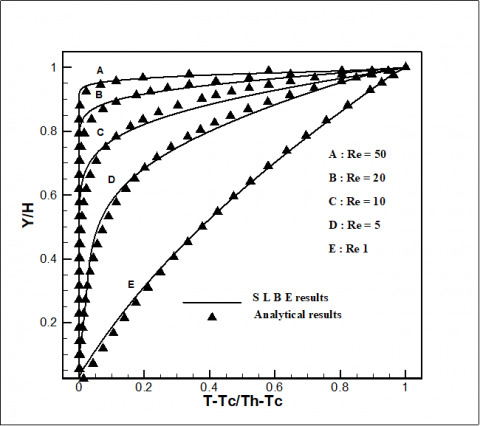(b) Temperature profile

Figure 3. Velocity and temperature profiles for different Reynolds number, SLBE and analytical simulations

The Figure 3(a) proves that when Re increases the porous media resistance to velocity fields becomes more important. The velocity layer thickness decreases by approaching to the moving wall. The Figure 3(b) shows that the increment of Reynolds number generates the decline of temperature. This is due to the strength convection in the channel when Re is large. More heat will be taken away by the flow.

The figure 3(b) presents a good agreement with asymptotic solution. For different Reynolds number the velocity and the temperature RMSE between the LBM results and the analytical values are established. The results are given in Table 5.

Table 5. Velocity and temperature root mean square error

 Reynolds Number Velocity Root Mean Square Error Temperature  Root Mean Square Error 1 0,029655 0,024606 5 0,049384 0,024375 10 0,037766 0,054756 20 0,029198 0,028888 50 0,030654 0,014704

## figure_4.pngFigure 4. Velocity and temperature profiles for different Darcy number and porosity, the solid lines are SLBE results and the symbols are analytical solutions; (a, c) velocity profile (b, d) temperature profile

The Figure 4(a) shows that the increment of $\mathrm{Da}$ is accompanied by the rise of the flow velocity. The flow attitude tends towards that of a free media. The velocity profile becomes more linear. The Figure 4(b) shows that there is an infinitesimal difference between the temperature profiles. This is interpreted by the negligible effect of horizontal flow. The heat transfer is vertical from bottom to top. For Reynolds and Darcy equal to 5 and 10-2, the porosity changes from 0.3 to 0.99. The Figure 4(c) shows that the porosity increment allows fluid flow readily. The porous medium drags decreases. The Figure 4(d) proves that the porosity change didn’t engender notified difference on the temperature profile.

The Table 6 describes the velocity and the temperature root mean square error between LBM results and the analytic values

Table 6. Root mean square at different porosity

 Porosity Velocity  Root Mean Square Error Temperature  Root Mean Square Error 0,99 0,024326 0,030568 0,5 0,032159 0,028316 0,3 0,036061 0,030438

4.3 GLBE and SLBE comparison

The inertia drags is modulated by Forchheimer term. Its effect on fluid flow and heat transfer is studied. So the previous study is performed using the GLBE We follow for each parameter the evolution of velocity and temperature values at a lattice node and the cross section (X=0.5). The GLBE and SLBE results are compared. The Figure 5 shows the velocity and temperature results at different dynamic parameter for a lattice node.

## figure_5.pngFigure 5. GLBE and SLBE results for different porosity, Reynolds and Darcy

The Figures 6 shows the velocity and temperature profiles at the lattice cross section $X=0.5$. The results are given at different Reynolds and Darcy number. Through the above Figures, the $D a$ or $R e$ increment hinders the ﬂow and the heat transfer. This is caused by the nonlinear drag force. This is observed also if the porosity decreases. Therefore, for small Darcy and Reynolds number the simulation can be restricted to the SLBE approach. For large value the Forchheimer term should not be neglected. The RMSE between the GLBE and SLBE determine the critical Reynolds and Darcy number for the selection of GLBE. Indeed, when $R M S E \leq 10^{-3}$ the SLBE is sufficient. In this case, the comparison shows that the critical values of respectively the Reynolds and Darcy number are less than 1 and 10-2.

## figure_6.pngFigure 6. Velocity and temperature profile using GLBE and SLBE for different Reynolds and for Darcy=0.1, (a, c) velocity profile (b, d) temperature profile

4.4 Thermal flow in porous plate with fixed wall

The geometry of the problem consists in a hot fluid forced to flow into a planar channel filled with porous media. The upper and lower plates are fixed and kept at a constant temperature $T_{c}$. On the left the distribution functions are established thanks to Zou and He conditions . In the upper and lower solid wall, the distribution functions are given by the no slip boundary conditions. For the thermal boundary, we resort to Dirichlet expressions (Eq(17)). The GLBE model is chosen for simulation. The velocity and the temperature profiles are plotted for different Reynolds number (Figure 7). The dynamic and thermal flow behavior inside the channel is described. The velocity and the temperature are plotted at different sections.

## figure_7.pngFigure 7. Velocity and temperature profiles for different Reynolds in different cross section, (a, d) velocity profile (b, c) temperature profile

The variation of Darcy number affects the velocity and the temperature. The results are presented in the Figure 8. When $D a$ is equal to 10-3 the flow is followed inside the porous channel. The temperature and the velocity at different cross sections are presented.

## figure_8.pngFigure 8. Velocity and temperature profiles for different Darcy number and at different cross sections, (a, c) velocity profile (b, d) temperature profile

Table 7. The evolution of the average Nusselt number and permeability at different porosity

 Reynolds number Darcy number porosity Average Nu Perme-ability 5 10-2 0.99 0.876 437.281 0.7 0.750 0.1717 0.5 0.631 0.022 0.1 0.516 0.00005

In these models, the solid fraction determines the local permeability at each node. Here, we measure the permeability of the different models by applying a pressure differential across a domain filled with nodes of a single solid fraction. The isotropic (thus scalar) intrinsic permeability $K$ is calculated from Darcy’s law.

$K=\frac{\varepsilon^{3} d_{p}^{2}}{150(1-\varepsilon)^{2}}$(22)

$d_{p}$: The solid particle diameter.

4.5 Heat transfer and fluid flow in porous media containing hot obstacle

The problem consists of a hot fluid flow in porous channel containing hot obstacle Figure 9.

## figure_9.pngFigure 9. Scheme of flow in the porous channel

The presence of the hot block requires the use of the Dirichlet boundaries for thermal conditions. For the dynamic conditions the Bounce back boundaries are applied. For all the simulation the previous circumstances are kept. The results at different factors are given by the following Figures.

The Figure 10 reports the sensibility to the porosity variation. For lower porosity the temperature of the fluid increases. This can be explained by the increment of the thermal conductivity by the porosity decrease. The increment of the porosity leads to a slight rise of velocity at the top and bottom of the block. The increment of the porosity makes easier for the fluid to vary its paths to the upper and bottom wall. Thus the velocity increases on the walls.

## figure_10.pngFigure 10. Temperature and velocity contours for different porosity respectively 0.5 and 0.9 at 6000 time step; (a, c) temperature (b, d) velocity contours

## figure_11.png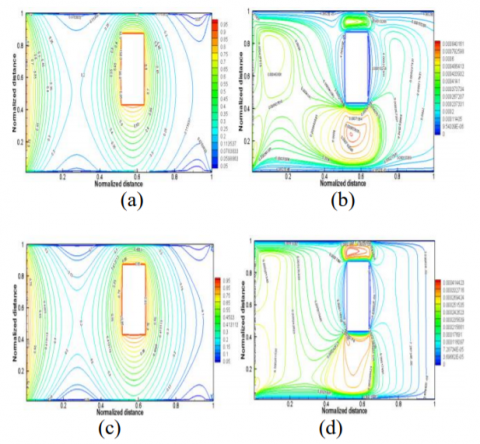Figure 11. Temperature and velocity contours for different Darcy number respectively 10-4 and 10-5 at 8000 time step; (a, c) temperature (b, d) velocity contours

Secondly the sensibility of the Darcy number is studied (Figure 11). Through this Figure, the Darcy variation affects the heat transfer and especially the velocity field. Their independence is due to constant properties of the flow. The increment of the Darcy generates an infinitesimal increase of the fluid temperature and the heat transfer. The velocity field increases due to the large permeability values.The velocity and temperature contours for different Reynolds number are plotted in Figure 12. As the Reynolds number increases the velocity of the flow increases. Consequently, the convective coefficient and the magnitude of heat transfer increase. For high Reynolds number the medium temperature wanes due to the decrease in the heat transfer process.

## figure_12a.png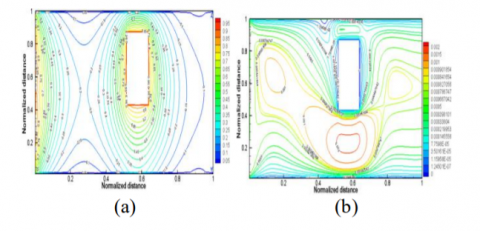## figure_12b.pngFigure 12. Temperature and velocity contours for different Reynolds number respectively 5 and 20 at 8000 time step; (a, c) temperature (b, d) velocity contours

## figure_13.png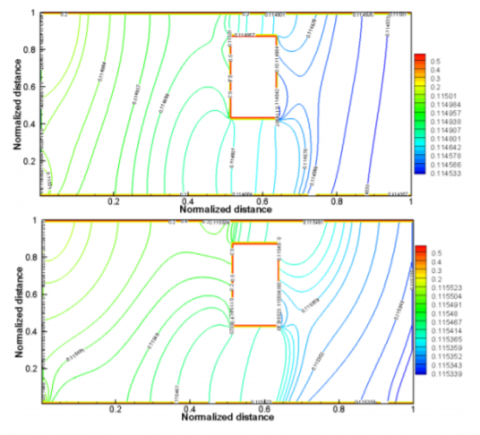Figure 13. Pressure contours at different Reynolds number respectively 20 and 5

The Figure 13 shows the pressure distribution in the porous medium at different Reynolds number. The high pressure region is significantly increased in upstream zone, which extends over obstacle. The velocity vector fields at different Reynolds number is given by the Figure 14. These results indicate that the obstacle strength plays an important role to increase the intensity of velocity magnitude in the obstacle bottom and top zone.

## figure_14.pngFigure 14. Velocity vectors field and vortices for different Reynolds number respectively 20 and 5

## figure_15.png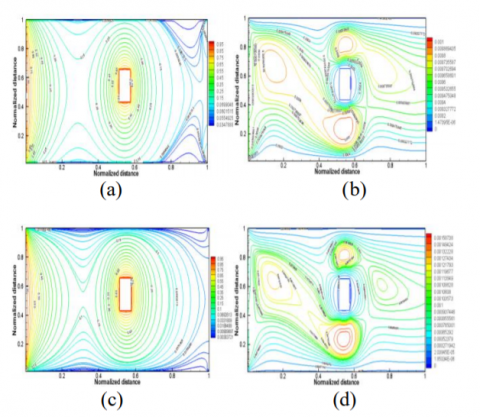Figure 15. Temperature and velocity contours at different Reynolds number respectively 5 and 20 at 8000 time step; (a, c) temperature (b, d) velocity contours

It is clear from the velocity vector plots in Figure 14 that the zone near obstacle will be a region of strong axial vortices, at least in cross flow, adjacent the obstacle there are small regions of concentrated vortices. The effect of the obstacle dimension and the position is studied for different Reynolds number. The length and width are 20 cm. It is placed in the channel center. Figure 15 shows the isotherms and the velocity contours. The obstacle suitable distances from the wall. Hence, the boundaries conditions do not affect the flow. The influence of the obstacle is well appeared. As a result, the dimensionless temperature and velocity contours are significantly different for these two cases with different obstacle dimensions

5. Conclusions

To overcome the insufficient of the Darcy equation at high speed two approaches are advanced. The Brinkman correction modulates the viscous stresses introduced by the solid boundary. Since convection is a boundary phenomenon this extension is significant. The other correction is the Forchheimer one. It introduces the nonlinear drags due to the solid matrix. The SLBE model is applied to simulate mixed convection with moving walls. Indeed, it is used to analyze the effect of dynamic parameter on velocity and temperature profiles. The flow is tracked at a lattice node and in a cross section. The results show an agreement with the analytic solution. SLBE allows also plotting the streamlines and the isotherms. The comparison, between GLBE and SLBE results, indicates that for small Reynolds and Darcy number we can limit to SLBE model. However, for large value the Forchheimer term should be incorporated and the GLBE is more suitable for simulation. The GLBE model is adopted to study the mixed convection in porous channel with fixed walls at different parameters. It’s also applied to reveal fluid flow and heat transfer over a hot solid block placed inside the channel. The temperature and the velocity contours at different parameters are plotted. This model can be developed to simulate convoluted phenomena in many industrial fields such as civil and mechanical engineering.

Nomenclature
 c Lattice spacing ci Discrete velocity for D2Q9 model c0 Coefficient for temporal velocity c1 Coefficient for temporal velocity f Density distribution function feq Density equilibrium distribution function F Total body force $F_{\varepsilon}$ Geometric factor G An external force g0 Gravity acceleration g Thermal distribution function geq Thermal equilibrium distribution function i Lattice index in the x direction j Lattice index in the y direction K Permeability (m2) k Effective thermal conductivity M Simulation value n The position on the right boundary p Pressure Pr Prandlt number T Fluid temperature Tk The temperature Tm Average temperature u Fluid velocity uin X- Component velocity in the inlet V0 Top wall velocity v0 Intial velocity z Total number of simulation value Greek letters $\alpha$ Thermal diffusivity $\beta$ Thermal expansion $\Gamma_{v}$ Thermal relaxation time $\delta t$ Time step $\theta$ Analytic or benchmark values v Viscosity (m2/s) ve Effective viscosity ρ Density  of medium (kg/m3) ρin Inlet density ρN Density of the upper plate $\sigma$ The ratio between $c_{p_{S}}\left(J \cdot k g^{-1} \cdot K^{-1}\right)$ and $C_{pf}$ $\Sigma$ Internal energy $\omega_i$ The weight coefficient i in the direction Subscripts i Discrete velocity direction in Inlet Superscript eq Equilibrium
References

 Nor Azwadi Che Sidik and Mohd Rosdzimin Abdul Rahman, “Cubic interpolated pseudo particle (CIP) - Thermal BGK lattice Boltzmann numerical scheme for solving incompressible thermal fluid flow problem,” Malaysian journal of mathematical sciences, vol. 3, no. 2, pp. 183-202, 2009.

 Nor Azwadi Che Sidik and Mohd Irwan Mohd Azmi, “Mesoscale numerical method for prediction of thermal fluid flow through porous media,” Wseas Transactions on Heat and Mass Transfer, vol. 7, no. 1, pp. 11-15, 2012.

 Igor Mele and Iztok Tiselj, “Lattice Boltzmann method,” Seminar, Faculty of mathematics and physics, Ljubljani Univ., Ljubljana, 2013. [Online]. Available: http://ma ja.fmf.uni-lj.si/seminar/ les/2012-2013/Lattice Boltzmann method.pdf.

 W. Regulski and J. Szumbarski, “Numerical simulation of conﬁned ﬂows past obstacles – the comparative study of Lattice Boltzmann and Spectral Element Methods,” Arch. Mech., vol. 64, no. 4, pp. 423–456, 2012.

 X. Jie, “A generalized Lattice-Boltzmann Model of fluid flow and heat transfer in porous media,” Master of Engineering, Dept Mec. Eng., Singapore Univ., Singapore, 2007. [Online]. Available: http://scholarbank.nus.edu.sg/bitstream/handle/10635/13351/Thesis%20of%20XIONG%20JIE.pdf?sequence=1.

 Takeshi Seta, Kazuyuki Kitano and Kenichi Okui, “Thermal lattice Boltzmann model for incompressible flows through porous media,” Journal of Thermal Science and Technology, vol. 1, no. 2, pp. 90-100, 2006. DOI: 10.1299/jtst.1.90.

 Zhaoli Guo and T. S. Zhao, “Lattice Boltzmann model for incompressible ﬂows through porous media,” Physical review E, vol. 66, 036304, pp. 1-9, 2002. DOI: 10.1103/PhysRevE.66.036304.

 M. Kaviany, “Laminar flow through a porous channel bounded by isothermal parallel plate,” International Journal of Heat and Mass Transfer, vol. 28, no. 4, pp. 851–858, 1985 DOI: 10.1016/0017-9310(85)90234-0.

 Neda Janzadeh and Mojtaba Aghajani Delavar, “Using lattice Boltzmann method to investigate the effects of porous media on heat transfer from solid block inside a channel,” Transport Phenomena in Nano and Micro Scales, vol. 1, no. 02, pp. 117-123, 2013.

 Nor Azwadi Che Sidik, Maysam Khakbaz, Leila Jahanshaloo, Syahrullail Samion and Amer Nordin Darus, “Simulation of forced convection in a channel with Nano fluid by the lattice Boltzmann method,” Nanoscale Res Lett, vol. 8, no. 1, pp. 1-8, 2013. DOI: 10.1186/1556-276X-8-178.

 Neda Janzadeh and Mojtaba Aghajani Delavar, “Numerical investigation of forced convection in a channel with solid block inside a square porous block,” Journal of energy, vol. 2013, ID 327179, pp. 1-8, 2013. DOI: 10.1155/2013/327179.

 Mousa Farhadi, Abbasali Abouei Mehrizi, Korush Sedighi and Hamid Hasanzadeh Afrouzi, “Effect of obstacle position and porous medium for heat transfer in an obstructed ventilated cavity,” Jurnal Teknologi (Sciences & Engineering), vol. 58, suppl. 2, pp. 59-64, 2012.

 M. Nazari, R. Mohebbi and M. H. Kayhani, “Power- Law fluid flow and heat transfer in a channel with a built-in porous square cylinder: lattice Boltzmann method,” Journal of non-Newtonian fluid mechanics, vol. 204, pp. 38-49, 2014. DOI: 10.1016/j.jnnfm.2013.12.002.

 Saida. Chatti, Chekib. Ghabi and Abdallah. Mhimid, “Effect of obstacle presence for heat transfer in porous channel,” Lecture Notes in Mechanical Engineering, vol. 789, pp. 823-832, 2015, DOI: 10.1007/978-3-319-17527-0_82.

 Ahmed Mahmoudi, Imen Mejri and Ahmed Omri, “Study of natural convection in a square cavity filled with nanofluid and subjected to a magnetic field,” International Journal of Heat And Technology, vol. 34, no. 1, pp. 73-79, 2016. DOI: 10.18280/ijht.340111.

 Takeshi Seta, “Lattice Boltzmann method for natural convection in anisotropic porous media,” Journal of Fluid Science and Technology, vol. 5, no. 3, pp. 585-602, 2010. DOI: 10.1299/jfst.5.585.

 ShouGuang Yao, XinWang Jia, AnJie Hu and RongJuan Li, “Analysis of Nano fluids Phase Transition in Pipe Using the Lattice Boltzmann Method,” International  Journal  Of  Heat  And  Technology, vol.33, no.2, 2015. DOI: 10.18280/ijht.330217.

 Zhaoli Guo and T. S. Zhao, “A lattice Boltzmann model for convection heat transfer in porous media,” Numerical Heat Transfer, Part B, vol. 47, no. 2, pp. 157–177, 2005. DOI: 10.1080/ 10407790590883405.

 A. A. Mohamad, “Isothermal Incompressible Fluid Flow,” in Lattice Boltzmann Method: Fundamentals and Engineering Applications with Computer Codes, Springer-Verlag London Limited, 2011, ch. 5, pp. 67-90, DOI: 10.1007/978-0-85729-455-5_5.